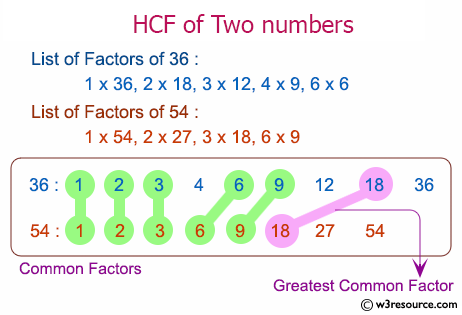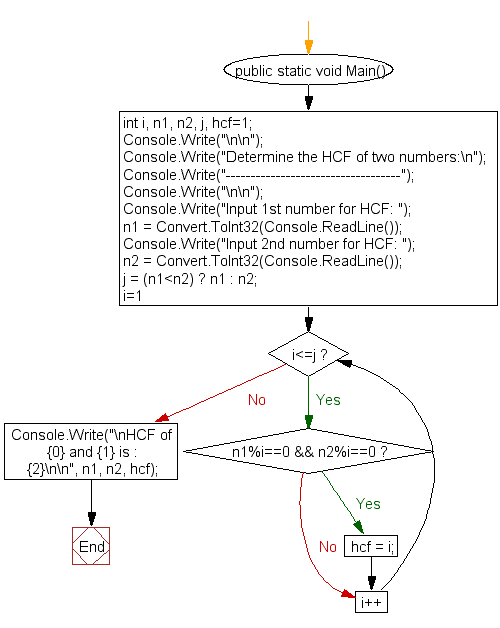﻿ C# - Determine the HCF of two numbers - w3resource# C# Sharp Exercises: Determine the HCF of two numbers

## C# Sharp For Loop: Exercise-43 with Solution

Write a C# Sharp program to find HCF (Highest Common Factor) of two numbers.Sample Solution:-

C# Sharp Code:

``````using System;
public class Exercise43
{
public static void Main()
{
int i, n1, n2, j, hcf=1;
Console.Write("\n\n");
Console.Write("Determine the HCF of two numbers:\n");
Console.Write("-----------------------------------");
Console.Write("\n\n");

Console.Write("Input 1st number for HCF: ");
Console.Write("Input 2nd number for HCF: ");
j = (n1<n2) ? n1 : n2;
for(i=1; i<=j; i++)
{
if(n1%i==0 && n2%i==0)
{
hcf = i;
}
}
Console.Write("\nHCF of {0} and {1} is : {2}\n\n", n1, n2, hcf);
}
}
```
```

Sample Output:

```Determine the HCF of two numbers:
-----------------------------------
Input 1st number for HCF: 10
Input 2nd number for HCF: 14
HCF of 10 and 14 is : 2
```

Flowchart:C# Sharp Code Editor:

Contribute your code and comments through Disqus.

What is the difficulty level of this exercise?

Test your Programming skills with w3resource's quiz.

﻿